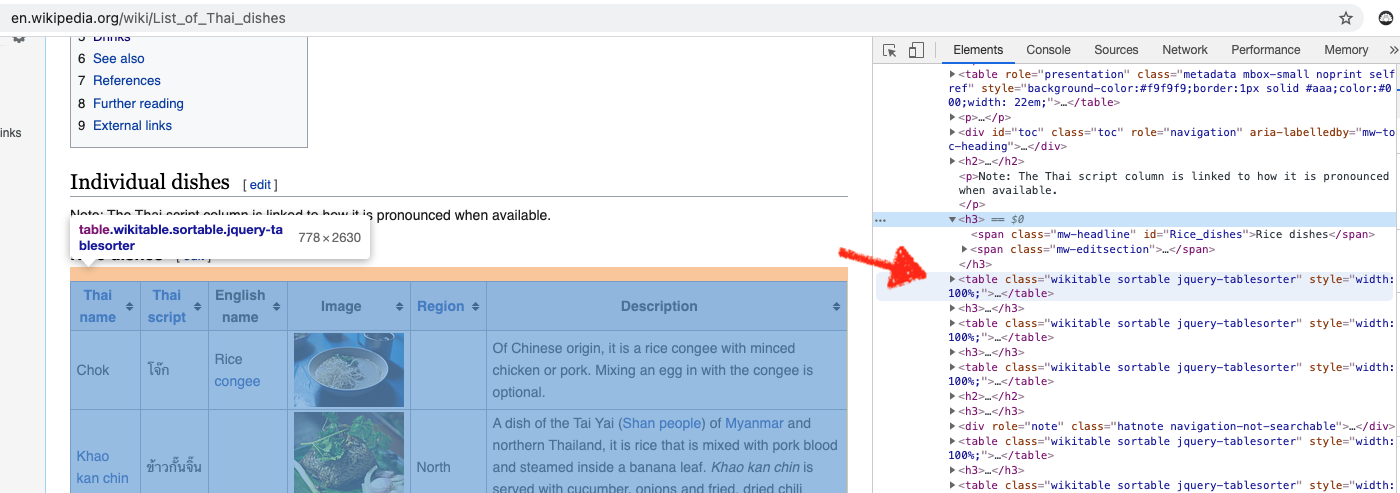# Paul Apivat Data Journey# Using Python to Scrape Thai Food Data

Photo by Alyssa Kowalski on Unsplash

### Overview

"Let's order Thai."

This has bugged me for years and is the genesis for this project.

People need to know they have other choices aside from Pad Thai. Pad Thai is one of 53 individual dishes and stopping there risks missing out on at least 201 shared Thai dishes (source: wikipedia).

This project is an opportunity to build a data set of Thai dishes by scraping tables off Wikipedia. We will use Python for web scraping and R for visualization. Web scraping is done in `Beautiful Soup` (Python) and pre-processed further with `dplyr` and visualized with `ggplot2`.

Furthermore, we'll use the `tidytext` package in R to explore the names of Thai dishes (in English) to see if we can learn some interest things from text data.

Finally, there is an opportunity to make an open source contribution.

The project repo is here.

### Exploratory Questions

The purpose of this analysis is to generate questions.

Because exploratory analysis is iterative, these questions were generated in the process of manipulating and visualizing data. We can use these questions to structure the rest of the post:

1. How might we organized Thai dishes?
2. What is the best way to organized the different dishes?
3. Which raw material(s) are most popular?
4. Which raw materials are most important?
5. Could you learn about Thai food just from the names of the dishes?

### Web Scraping

We scraped over 300 Thai dishes. For each dish, we got:

• Thai name
• Thai script
• English name
• Region
• Description

First, we'll use the following Python libraries/modules:

``````import requests
from bs4 import BeautifulSoup
import urllib.request
import urllib.parse
import urllib.error
import ssl
import pandas as pd
``````

We'll use `requests` to send an HTTP requests to the wikipedia url we need. We'll access network sockets using 'secure sockets layer' (SSL). Then we'll read in the html data to parse it with Beautiful Soup.

Before using Beautiful Soup, we want to understand the structure of the page (and tables) we want to scrape under inspect element on the browser (note: I used Chrome). We can see that we want the `table` tag, along with `class` of wikitable sortable.The main function we'll use from Beautiful Soup is `findAll()` and the three parameters are `th` (Header Cell in HTML table), `tr` (Row in HTML table) and `td` (Standard Data Cell).

First, we'll save the table headers in a list, which we'll use when creating an empty `dictionary` to store the data we need.

``````header = [item.text.rstrip() for item in all_tables.findAll('th')]

table = dict([(x, 0) for x in header])
``````

Initially, we want to scrape one table, knowing that we'll need to repeat the process for all 16 tables. Therefore we'll use a nested loop. Because all tables have 6 columns, we'll want to create 6 empty lists.

We'll scrape through all table rows `tr` and check for 6 cells (which we should have for 6 columns), then we'll append the data to each empty list we created.

``````# loop through all 16 tables
a = [0, 1, 2, 3, 4, 5, 6, 7, 8, 9, 10, 11, 12, 13, 14, 15]

# 6 empty list (for 6 columns) to store data
a1 = []
a2 = []
a3 = []
a4 = []
a5 = []
a6 = []

# nested loop for looping through all 16 tables, then all tables individually
for i in a:
for row in all_tables[i].findAll('tr'):
cells = row.findAll('td')
if len(cells) == 6:
a1.append([string for string in cells.strings])
a2.append(cells.find(text=True))
a3.append(cells.find(text=True))
a4.append(cells.find(text=True))
a5.append(cells.find(text=True))
a6.append([string for string in cells.strings])
``````

You'll note the code for `a1` and `a6` are slightly different. In retrospect, I found that `cells.find(text=True)` did not yield certain texts, particularly if they were links, therefore a slight adjustment is made.

The strings tag returns a `NavigableString` type object while text returns a `unicode` object (see stack overflow explanation).

After we've scrapped the data, we'll need to store the data in a `dictionary` before converting to `data frame`:

``````# create dictionary
table = dict([(x, 0) for x in header])

# append dictionary with corresponding data list
table['Thai name'] = a1
table['Thai script'] = a2
table['English name'] = a3
table['Image'] = a4
table['Region'] = a5
table['Description'] = a6

# turn dict into dataframe
df_table = pd.DataFrame(table)
``````

For `a1` and `a6`, we need to do an extra step of joining the strings together, so I've created two additional corresponding columns, `Thai name 2` and `Description2`:

``````# Need to Flatten Two Columns: 'Thai name' and 'Description'
# Create two new columns
df_table['Thai name 2'] = ""
df_table['Description2'] = ""

# join all words in the list for each of 328 rows and set to thai_dishes['Description2'] column
# automatically flatten the list
df_table['Description2'] = [
' '.join(cell) for cell in df_table['Description']]

df_table['Thai name 2'] = [
' '.join(cell) for cell in df_table['Thai name']]
``````

After we've scrapped all the data and converted from `dictionary` to `data frame`, we'll write to CSV to prepare for data cleaning in R (note: I saved the csv as thai_dishes.csv, but you can choose a different name).

For more content on data science, R, Python, SQL and more, find me on Twitter.